# @微信官方给不了的圣诞帽，Python和OpenCV给你（附代码）

• OpenCV（毕竟我们主要的内容就是OpenCV...）
• dlib（前一篇文章刚说过，dlib的人脸检测比OpenCV更好用，而且dlib有OpenCV没有的关键点检测。）r,g,b,a = cv2.split(hat_img)
rgb_hat = cv2.merge((r,g,b))
cv2.imwrite("hat_alpha.jpg",a)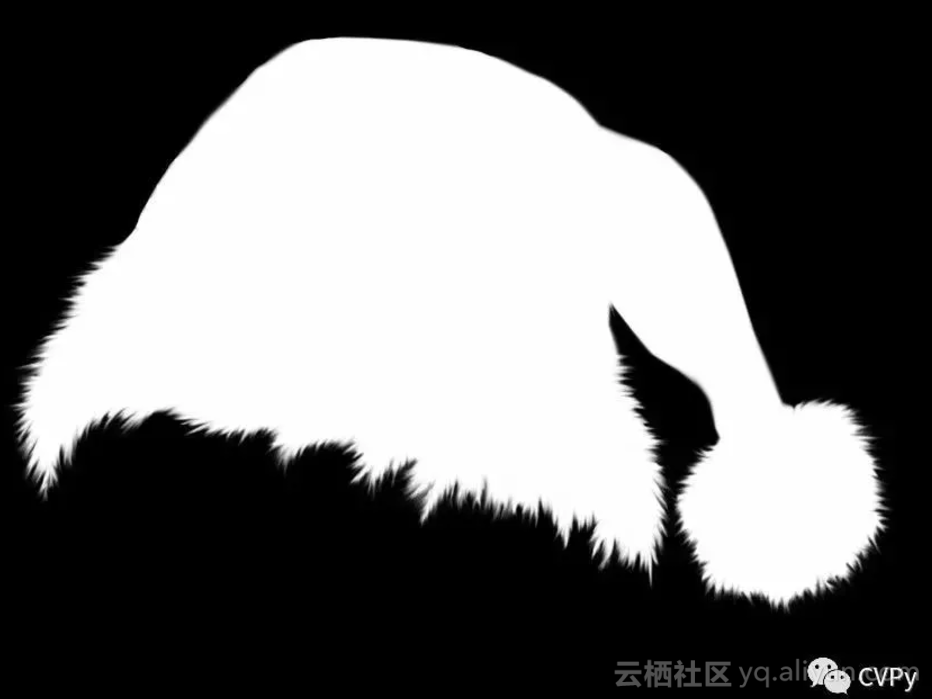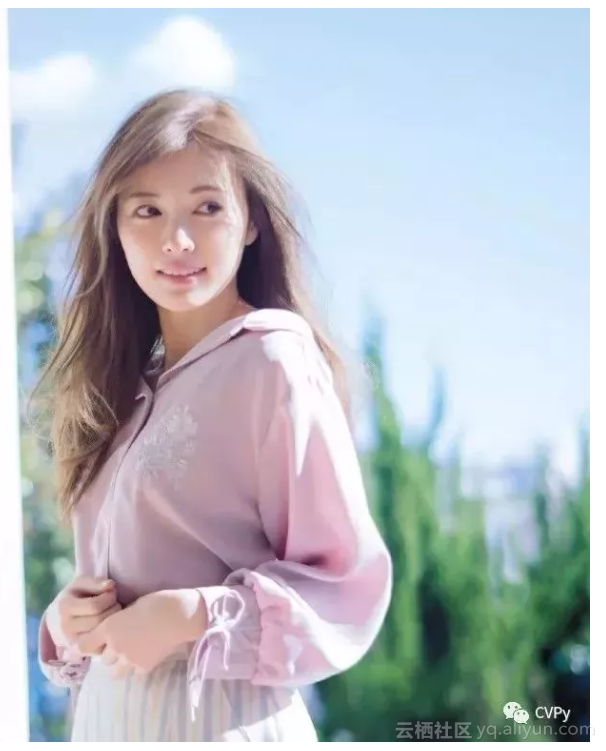# dlib人脸关键点检测器

predictor_path = "shape_predictor_5_face_landmarks.dat"

predictor = dlib.shape_predictor(predictor_path)

# dlib正脸检测器

detector = dlib.get_frontal_face_detector()

# 正脸检测

dets = detector(img, 1)

# 如果检测到人脸

if len(dets)>0:

for d in dets:

x,y,w,h = d.left(),d.top(), d.right()-d.left(), d.bottom()-d.top()

# x,y,w,h = faceRect

cv2.rectangle(img,(x,y),(x+w,y+h),(255,0,0),2,8,0)

# 关键点检测，5个关键点

shape = predictor(img, d)

for point in shape.parts():

cv2.circle(img,(point.x,point.y),3,color=(0,255,0))

cv2.imshow("image",img)

cv2.waitKey()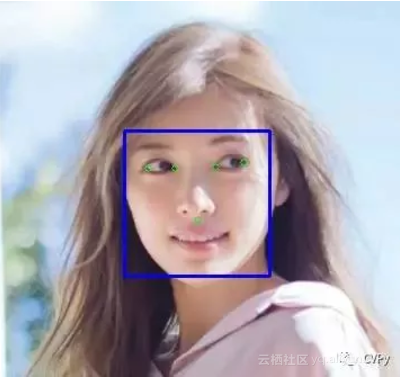### 三、调整帽子大小

# 选取左右眼眼角的点

point1 = shape.part(0)

point2 = shape.part(2)

# 求两点中心

eyes_center = ((point1.x+point2.x)//2,(point1.y+point2.y)//2)

# cv2.circle(img,eyes_center,3,color=(0,255,0))

# cv2.imshow("image",img)

# cv2.waitKey()

#  根据人脸大小调整帽子大小

factor = 1.5

resized_hat_h = int(round(rgb_hat.shape*w/rgb_hat.shape*factor))

resized_hat_w = int(round(rgb_hat.shape*w/rgb_hat.shape*factor))

if resized_hat_h > y:

resized_hat_h = y-1

# 根据人脸大小调整帽子大小

resized_hat = cv2.resize(rgb_hat,(resized_hat_w,resized_hat_h))

#### 四、提取帽子和需要添加帽子的区域

# 用alpha通道作为mask

mask = cv2.resize(a,(resized_hat_w,resized_hat_h))

mask_inv =  cv2.bitwise_not(mask)

# 帽子相对与人脸框上线的偏移量

dh = 0

dw = 0

# 原图ROI

# bg_roi = img[y+dh-resized_hat_h:y+dh, x+dw:x+dw+resized_hat_w]

bg_roi = img[y+dh-resized_hat_h:y+dh,(eyes_center-resized_hat_w//3):(eyes_center+resized_hat_w//3*2)]

# 原图ROI中提取放帽子的区域

bg_roi = bg_roi.astype(float)

mask_inv = cv2.merge((mask_inv,mask_inv,mask_inv))

alpha = mask_inv.astype(float)/255

# 相乘之前保证两者大小一致（可能会由于四舍五入原因不一致）

alpha = cv2.resize(alpha,(bg_roi.shape,bg_roi.shape))

# print("alpha size: ",alpha.shape)

# print("bg_roi size: ",bg_roi.shape)

bg = cv2.multiply(alpha, bg_roi)

bg = bg.astype('uint8')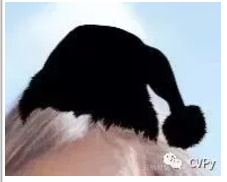# 提取帽子区域

hat = cv2.bitwise_and(resized_hat,resized_hat,mask = mask)#### 五、添加圣诞帽

# 相加之前保证两者大小一致（可能会由于四舍五入原因不一致）

hat = cv2.resize(hat,(bg_roi.shape,bg_roi.shape))

# 两个ROI区域相加

add_hat = cv2.add(bg,hat)

# cv2.imshow("add_hat",add_hat)

# 把添加好帽子的区域放回原图

img[y+dh-resized_hat_h:y+dh,(eyes_center-resized_hat_w//3):(eyes_center+resized_hat_w//3*2)] = add_hat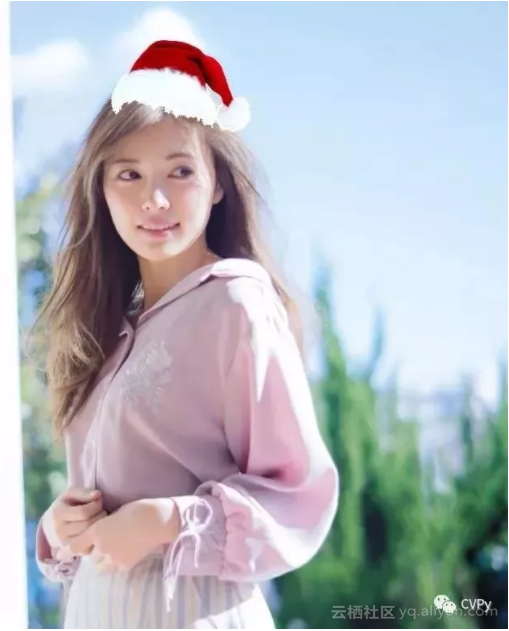https://github.com/LiuXiaolong19920720/Add-Christmas-Hat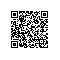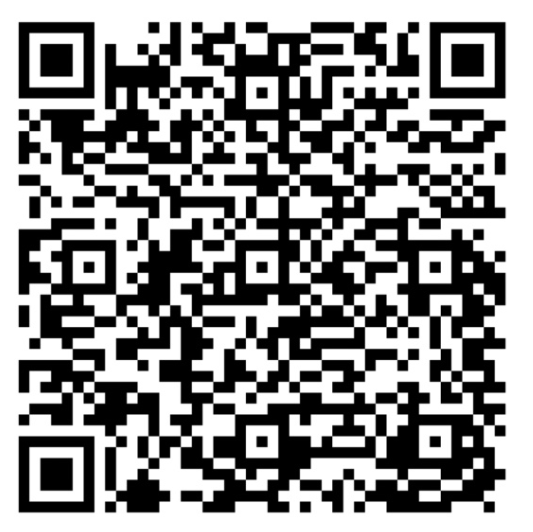使用钉钉扫一扫加入圈子
+ 订阅